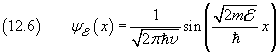## 12. Wave function of a free particle.

The description of the quantum mechanical state of a free particle may be derived from the results got in section 8 as asymptotic case with L (distance between walls) approaching the infinity. Obviously now we can't apply the same boundary conditions as there but, like there, we can assume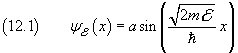.

Instead to the energy, we can refer the wave function to the momentum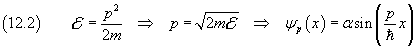The wave amplitude is now expressed as α because it depends on the normalization procedure of the function.

In order to normalize the function ψp we cannot simply make equal to 1 the integral of the square of the equation (12.2) from -∞ to +∞, because this integral, which is essentially a sum of an infinite number of positive quantities, diverges. Since ψp is a continuous function of a continuous variable p, we will integrate the square of the integral intermediate value of ψp on the interval [p0 , p0+Δp],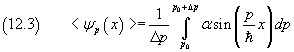The integration gives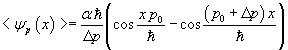Considering progressive and regressive waves the normalization condition results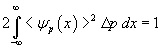The integration gives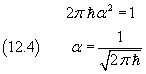Therefore the equation (12.2) can be more fully written as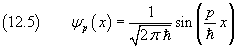To write the wave equation in terms of energy, we change the differential in (12.3)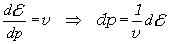so that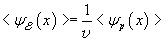This gives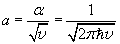.

In conclusion, the wave equation of a free particle with energy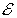is